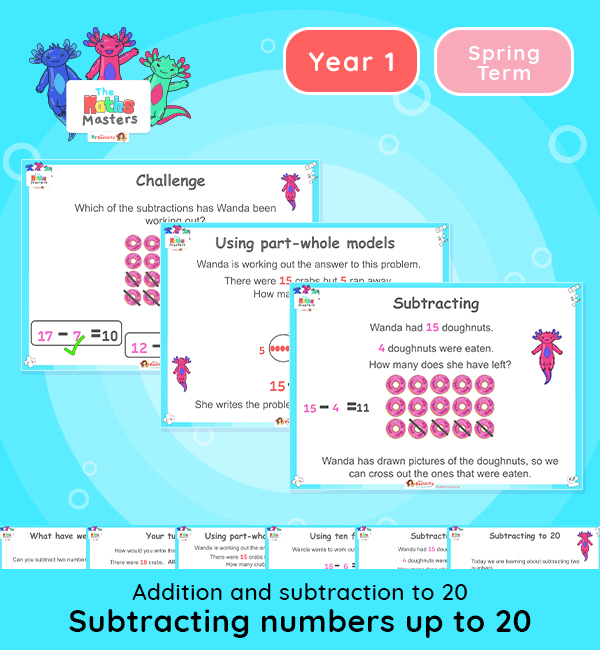# Year 1 | Subtracting Numbers up to 20 Lesson Presentation## White Rose Year 1 addition and subtraction lesson plans

Aligned with the White Rose Maths scheme of work, this Year 1 | Subtracting Numbers up to 20 Lesson Presentation is fully editable, and is designed for the Year 1 maths curriculum covering the following maths objectives for the autumn term:

Topic: Addition and subtraction to 20

This lesson practises subtracting numbers within 20 without crossing ten. It will recap on the methods of taking away: using counters, part-whole models, and crossing out pictures to find the answer.

White Rose Maths Small Steps: Subtraction (not crossing ten)

NC Links: Read, write and interpret mathematical statements involving addition, subtraction and equals signs. *Add and subtract one-digit and two-digit numbers to 20, including zero. *Solve one step problems that involve addition and subtraction using concrete objects and pictorial representations , and missing number problems.

TAF Statements: Working towards: Add and subtract one digit number explaining their method verbally, in pictures, or using apparatus.

Working At: Recall all the number bonds to and within 10 and use these to reason with and calculate bonds to and within 20, recognising other associated additive relationships.

Greater Depth – Use reasoning about number and relationships to solve more complex problems and explain their thinking.

Ready-to-progress criteria:  1AS-2 Read, write and interpret equations containing addition, subtraction and equals symbols and relate additive expressions and equations to real-life contexts.

Previous experience: Devise and record number stories, using pictures, numbers and symbols.

## Recently Viewed